# Solve Rational Inequalities Examples With Solutions

Rational Inequalities are solved in the examples below. Knowing that the sign of an algebraic expression changes at its zeros of odd multiplicity, solving an inequality may be reduced to finding the sign of an algebraic expression within intervals defined by the zeros of the expression in question.

## Solve the following inequalities

### Questions a)### Solution

We first arrange the zeros of the numerator and the denominator, of the rational expression on the left of the inequality symbol, on the number line, from smallest to the largest as follows.
 -∞ - 1 2 +∞

Select a value of x in any of the intervals and use it to find the sign of the rational expression. Example for x = -3 in the interval (-∞ , -1), the rational expression (x - 2)/(x + 1) = (- 3 - 2)/(- 3 + 1) = 5 / 2. Hence the rational expression (x - 2)/(x + 1) is positive on the interval (-∞ , -1) .
 -∞ + - 1 2 +∞
The zeros -1 and 2 are of odd multiplicity and therefore the sign of the expression (x - 2)/(x + 1) will change at both zeros as we go from on interval to another. Hence the signs of the expression (x - 2)/(x + 1) as we go from left to right are
 -∞ + - 1 - 2 + +∞
The solution set of the inequality is given by the union of all intervals where (x - 2)/(x + 1) is positive or equal to 0. Hence the solution set for the above inequality, in interval notation, is given by:
(-∞ , -1) ∪ [ 2 , +∞)

### Questions b)### Solution

We first rewrite the given inequality with the right side equal to zero.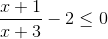Use x + 3 as a common denominator to rewrite the left side of the inequality as a single rational expressions as follows.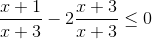add the two rational expressions to obtain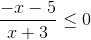We arrange the zeros of the numerator and the denominator on the number line from smallest to the largest as follows.
 -∞ - 5 - 3 +∞

Select a value of x in the interval (-∞ , - 5) and use it to find the sign of the rational expression. Example for x = - 6, the rational expression (-x - 5)(x + 3) = (6 - 5)/(- 6 + 3) = -1 / 3. Hence the rational expression (-x - 5)(x + 3) is negative on the interval (-∞ , - 5) .
 -∞ - - 5 - 3 +∞

The zeros - 5 and - 3 are of odd multiplicity and therefore the sign of (-x - 5)(x + 3) will change at both zeros. Hence the signs of the expression (-x - 5)(x + 3) as we go from left to right are
 -∞ - - 5 + - 3 - +∞

The solution set of the inequality is given by the union of all intervals where (-x - 5)(x + 3) is negative or equal to 0. Hence the solution set for the above inequality, in interval notation, is given by:
(-∞ , - 5] ∪ ( - 3 , +∞)

### Questions c)### Solution

We need to find the zeros of the numerator and denominator by factoring (or any other method). Factor the numerator and the denominator.
4 x
2 + 5x - 9 = (4x + 9)(x - 1) and x 2 - x - 6 = (x - 3)(x + 2)
Rewrite the given inequality as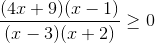Arrange the zeros of the numerator and the denominator on the number and in order from smallest to the largest as follows.
 -∞ - 9 / 4 - 2 1 3 +∞

Select a value of x in the interval (-∞ , - 9/4) and use it to find the sign of the rational expression. Example for x = - 3, the rational expression ( (4x + 9)(x - 1) ) / ( (x - 3)(x + 2) ) = 2. Hence the rational expression ( (4x + 9)(x - 1)) / ( (x - 3)(x + 2) ) is positive on the interval (-∞ , - 9/4) .
All the zeros are of odd multiplicity and therefore the sign of ( (4x + 9)(x - 1) ) / ( (x - 3)(x + 2) ) will change at all zeros. Hence the signs of the expression ( (4x + 9)(x - 1) ) / ( (x - 3)(x + 2) ) as we go from left to right are
 -∞ + - 9 / 4 - - 2 + 1 - 3 + +∞

The solution set of the inequality is given by the union of all intervals where ( (4x + 9)(x - 1) ) / ( (x - 3)(x + 2) ) is positive or equal to 0. Hence the solution set for the above inequality, in interval notation, is given by:
(-∞ , - 9/4] ∪ ( - 2 , 1] ∪ (3 , +∞)

### Questions d)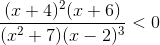### Solution

 -∞ - 6 - 4 2 +∞
Select a value of x in the interval (-∞ , - 6) and use it to find the sign of the rational expression. Example for x = - 7, the rational expression ( (x+4) 2(x+6) ) / ( (x 2+7)(x-2) 3) = 1/4536. Hence the rational expression ( (x+4) 2(x+6) ) / ( (x 2+7)(x-2) 3) is positive on the interval (-∞ , - 6) .
The sign of ( (x+4) 2(x+6) ) / ( (x 2+7)(x-2) 3) will change at the zeros -6 and 2 because they are of odd multiplicity. The sign of ( (x+4) 2(x+6) ) / ( (x 2+7)(x-2) 3) will not change at - 4 because it is a zero of even multiplicity. Hence the signs of the expression ( (x+4) 2(x+6) ) / ( (x 2+7)(x-2) 3) as we go from left to right are
 -∞ + - 6 - - 4 - 2 + +∞

The solution set of the inequality is given by the union of all intervals where ( (x+4) 2(x+6) ) / ( (x 2+7)(x-2) 3) is negative. Hence the solution set for the above inequality, in interval notation, is given by:
(- 6 , - 4) ∪ ( - 4 , 2)

### Questions e)Solution:
Factor the numerator and the denominator of the given inequality.
x
3 + 1 = (x + 1)(x 2 - x + 1) and x 2 - 9 = (x - 3)(x + 3)
Rewrite the given inequality as followsThe rational expression on the left side of the inequality has the zeros: - 3, - 1 and 3 ( x 2 - x + 1 has no real zeros). Arrange all the three zeros on the number and in order from smallest to the largest as follows.
 -∞ - 3 - 1 3 +∞

Select a value of x in the interval (-∞ , - 3) and use it to find the sign of the rational expression. Example for x = - 4, the rational expression ( x 3 + 1) / ( (x 2 - 9) ) = - 9. Hence the rational expression on the left side of the given inequality is negative on the interval (-∞ , - 3) .
The sign of the rational expression on the left side of the given inequality will change at the zeros - 1 and 3 because the are of odd multiplicity. Hence the signs of the expression rational expression as we go from left to right are
 -∞ - - 3 + - 1 - 3 + +∞

The solution set of the inequality is given by the union of all intervals where rational expression on the left side of the given inequality is negative. Hence the solution set for the above inequality, in interval notation, is given by:
(-∞ , - 3) ∪ ( - 1 , 3)

### Questions f)### Solution

We first rewrite the given inequality with the right side equal to zero.Find the LCD (least common denominator) of (x - 2)(x + 3) and x - 2 which is (x - 2)(x + 3).
Rewrite with common LCD.Add the rational expressions on the left and simplify.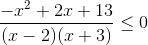The rational expression on the left side of the inequality has the zeros at : - 3, 1 - √14 , 2 and 1 + √14. Arrange all the zeros on the number and in order from smallest to the largest as follows.
 -∞ - 3 1 - √14 2 1 + √14 +∞

Select a value of x in the interval (-∞ , - 3) and use it to find the sign of the rational expression. Example for x = - 4, the rational expression (-x 2 + 2x + 13) / ( (x-2)(x+3) ) = -11/6. Hence the rational expression on the left side of the given inequality is negative on the interval (-∞ , - 3) .
The sign of the rational expression on the left side of the given inequality will change at all the zeros because they all have odd multiplicity. Hence the signs of the expression rational expression as we go from left to right are
 -∞ - - 3 + 1 - √14 - 2 + 1 + √14 - +∞

The solution set of the inequality is given by:
(-∞ , - 3) ∪ [ 1 - √14 , 2) ∪ [ 1 + √14 , +∞)

### Questions g)

Solve graphically### Solution

Graph the two sides of the inequality and approximate the coordinates of the points of intersection of the two graphs; then determine the intervals over which the (x + 2)/(x - 1) is greater than or equal to ln(x + 1).ln(x + 1) is defined for x > - 1 hence any solution set must satisfy the condition x > - 1. (x + 2)/(x - 1) has a vertical asymptote at x = 1. From the two graphs, (x + 2)/(x - 1) (green) is greater than or equal to ln(x + 1) (red) for
(- 1, -0.59] ∪ (1 , 4.88]